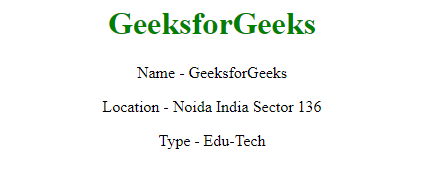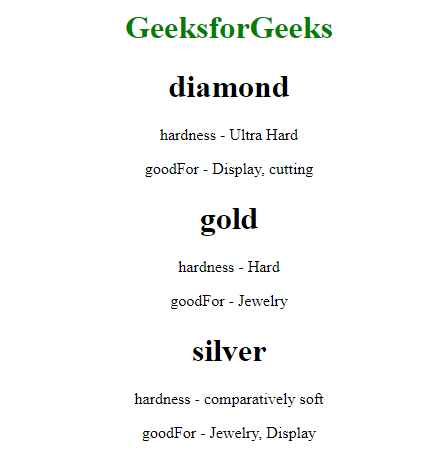Related Articles

# How to iterate over the keys and values with ng-repeat in AngularJS ?

• Last Updated : 22 Nov, 2019

The task is to iterate over a JS object (its keys and values) using the ng-repeat directive. This can be done using parenthesis in the ng-repeat directive to explicitly ask for a key-value pair parameter from angularJS. Here the variable key contains the key of the object and value contains the value of the object.

Syntax:

`<element ng-repeat="(key, value) in JSObject"> Contents... </element>`

Example 1: In this example, we will simply display all the keys and values of a JS object using ng-repeat. In first iteration, key = name and value = “GeeksforGeeks”. In 2nd iteration, key = location and value = “Noida India Sector 136″…This keeps on iterating until all the keys and their values are covered at least once similar to a for-each loop.

• Program:
 ```<``html` `ng-app``=``"myApp"``>`` ` `<``head``>``    ``<``script` `src``=``"https://ajax.googleapis.com/ajax/libs/angularjs/1.7.8/angular.min.js"``>``    `````` ` `<``body` `ng-controller``=``"MyController"``>``    ``<``center``>``        ``<``h1` `style``=``"color: green;"``>``            ``GeeksforGeeks``        ````        ``<``div` `ng-repeat``=``"(key, value) in gfg"``>``           ` `            ````            ``<``p``>{{key}} - {{value}}``        ````    ``````<``script` `type``=``"text/javascript"``>``    ``var myApp = angular.module('myApp', []);``    ``myApp.controller('MyController', ['\$scope', function(\$scope) {``        ``\$scope.gfg = {``            ``Name: "GeeksforGeeks",``            ``Location: "Noida India Sector 136",``            ``Type: "Edu-Tech",``        ``}``    ``}]);```` ` ``
• Output: On loading the page, we see that all the key-value pairs of the objects are already listed there. This is because the ng-repeat is called on load as the HTML gets loaded.Example 2: In this example, we will loop over a nested object using ng-repeat directive. In the first iteration, key = diamond and value = {hardness:”Ultra Hard”, goodFor:”Display, cutting”} in the next iteration key = gold and value is its respective object. This keeps on iterating like a for-each loop over the key-value pairs of the object materials.

• Program:
 ```<``html` `ng-app``=``"myApp"``>`` ` `<``head``>``    ``<``script` `src``=``"https://ajax.googleapis.com/ajax/libs/angularjs/1.7.8/angular.min.js"``>``    `````` ` `<``body` `ng-controller``=``"MyController"``>``    ``<``center``>``        ``<``h1` `style``=``"color: green;"``>``            ``GeeksforGeeks``        ````        ``<``div` `ng-repeat``=``"(key, value) in materials"``>`` ` `            ``<``h1``>{{key}}``            ``<``div` `ng-repeat``=``"(key1, value1) in value"``>`` ` `                ````                ``<``p``>{{key1}} - {{value1}}``            ````        ````    ``````<``script` `type``=``"text/javascript"``>``    ``var myApp = angular.module('myApp', []);``    ``myApp.controller('MyController', ['\$scope', function(\$scope) {``        ``\$scope.materials = {``            ``diamond: {``                ``hardness: "Ultra Hard",``                ``goodFor: "Display, cutting"``            ``},``            ``gold: {``                ``hardness: "Hard",``                ``goodFor: "Jewelry"``            ``},``            ``silver: {``                ``hardness: "comparatively soft",``                ``goodFor: "Jewelry, Display"``            ``}``        ``}``    ``}]);```` ` ``
• Output:My Personal Notes arrow_drop_up